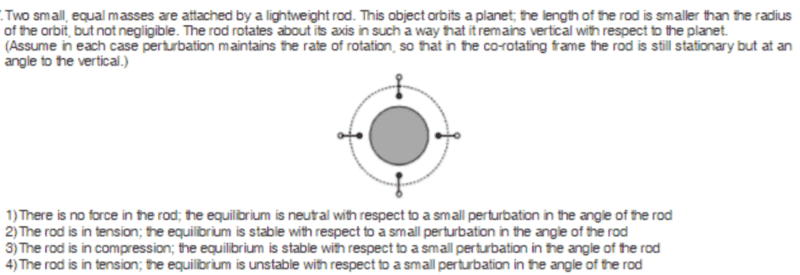# Equilibrium of an object orbiting around a planet

## Homework Statement## The Attempt at a Solution

Honestly , I have not been able to understand the question . I am totally clueless about the part written in parenthesis .

All I understand is that since the length of the rod is not negligible ,value of gravity at the location of the two masses is different .

I would really appreciate if someone could help me understand the problem statement and nudge me in the right direction .

Thank you .

#### Attachments

The parenthesis means this:
If something disturbs the rate of rotation of the rod, the angular momentum would to a first order be conserved and the rod could rotate to a point where it is for example parallel to the surface of the planet, in which case you have something totally different. They want the angular momentum of the rod to be fixed. This is not entirely unreasonable - the moon is also gravity-locked in a similar way. This should also tell you that the situation is probably stable. You already guessed correctly that the gravity is different and that the rod should then probably be in tension.

Now comes the hardest part: Why is the situation stable?
What I would do is write down an energy balance for a slightly rotated rod and see if the perpendicular state has the lowest energy. Hint: You probably need spherical/cylindrical coordinates.

The first thing I would like to understand is how the rod is able to remain vertical at all times . For this to happen , the farther mass ( say A ) should be travelling at a higher speed ( so as to cover larger diatance ) than the closer mass( say B ) . I am thinking of how a satellite in orbit of larger radius has smaller speed .

This is a good question. But you need to think of it in broader terms. Mass B feels a larger gravitational attraction and it is too slow for its orbit. So there's a slight pull inward. Same with A and outward. There's apparently no torque acting on the system, just tension. Now the actual question that you need to answer is this: If you're putting the rod slightly off from the vertical line, there will be some torque. Is it pulling the system back to vertical, so that the configuration is stable? Or is it pulling the system away from that state, so that it is unstable?

Mass B feels a larger gravitational attraction and it is too slow for its orbit. So there's a slight pull inward.

Sorry . Please explain this .

Sorry . Please explain this .

So, if we're talking about circular orbits you have usually the condition that the gravitationfal force is equal to the centripetal force

$$F_G=GMm/r^2=mv^2/r=F_r$$

The object which we have here is in orbit, which means that the center of mass is at position r and has the velocity v.
The rod is at all times pointing in the radial direction. B is at the position r-L/2. The speed is also different. The rotation period stays the same, but the radius changes.
Now $$F_{G,B}-F_{r,B}$$ is not zero. You can calculate how large it is, and if the gravitational force or the centripetal force is larger for B, so if it is pulled inwards or pushed outwards.